## What Is the Declining Balance Method of Assets Depreciation?

Under the declining balance method, depreciation is charged on the book value of the asset and the amount of depreciation decreases every year.

## Declining Balance Method: Explanation

As an alternative to systematic allocation schemes, several declining balance methods for calculating depreciation expenses have been developed.

They determine the annual charge by multiplying a percentage rate by the book value of the asset (not the depreciable basis) at the beginning of the year.

Because the book value declines as the asset ages and the rate stays constant, the depreciation charge falls each year.

Financial accounting applications of declining balance are often linked to income tax regulations, which allow the taxpayer to compute the annual rate by applying a percentage multiplier to the straight-line rate.

According to the type of property, the multiplier assumes a value such as 1.25, 1.50, or 2.00; when the multiplier is 2.00, the method is known as double-declining. The rates applied in different situations are shown below: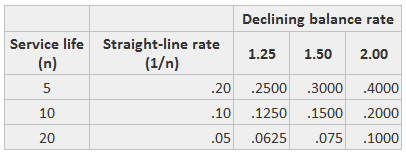Under the declining balance methods, the asset’s salvage value is used as the minimum book value; the total lifetime depreciation is thus the same as under the other methods.

The arbitrary rates used under the tax regulations often result in assigning depreciation to more or fewer years than the service life.

## Example

Under the declining balance method, yearly depreciation is calculated by applying a fixed percentage rate to an asset’s remaining book value at the beginning of each year.

Because twice the straight-line rate is generally used, this method is often referred to as double-declining balance depreciation.

For example, suppose that a piece of equipment has a five-year life. This results in an annual straight-line percentage rate of 20% (1 / 5 = 20%).

The double-declining balance rate is 40% (2 x 20%). This rate is applied to the asset’s remaining book value at the beginning of each year.

When applying the double-declining balance method, the asset’s residual value is not initially subtracted from the asset’s acquisition cost to arrive at a depreciable cost.

By contrast, the opposite is true when applying the straight-line method, the unit-of-production method, and the sum-of-the-years-digits method.

Residual value is considered only in the last year of the asset’s life. This is when that year’s depreciation is limited to the amount that will reduce the asset’s book value to its residual value.

These points are illustrated in the following schedule, which shows yearly depreciation calculations for the equipment in this example.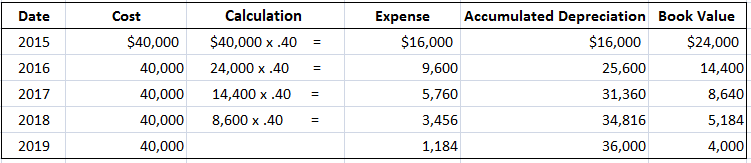* The depreciation expense in 2019 is the amount required to reduce the equipment’s book value to its residual value of \$4,000 (\$5,184 – \$4,000 = \$1,184).

Partial-year depreciation also can be calculated using the declining balance method.

For example, if the equipment in the above case is purchased on 1 October rather than 2 January, depreciation for the period between 1 October and 31 December is (\$16,000 x 3/12).

In the second year, depreciation is calculated in a regular way by multiplying the remaining book value of \$36,000 (\$40,000 — \$4,000) by 40%.

In the above example, we assumed a depreciation rate equal to twice the straight-line rate. However, many firms use a rate equal to 1.5 times the straight-line rate. This is called 150% declining balance depreciation.

150% declining balance depreciation is calculated in the same manner as is double-declining-balance depreciation, except that the rate is 150% of the straight-line rate.

## Advantages of the Declining Balance Method

1. Equal Burden: The method creates a burden to use assets more in each subsequent year. The amount of depreciation continues to decrease for each subsequent year, whereas the charge for repairs increases alongside this.

Thus, an increase in the cost of repairs of each subsequent year is compensated by a decrease in the amount of depreciation for each subsequent year.

2. Simple Method: An important strength of the declining balance method is that it is simple to understand and easy to follow.

3. Suitable for Tax Authorities: The method is recognized and accepted by income tax authorities.

## Disadvantages of Declining Balance Method

1. No Zero Value: The value of assets cannot be brought down to zero under this method.

2. Rate Determination Is Not Easy: Determining suitable depreciation rates is different and more complex compared to the fixed installment method.

## Formula

Under the declining balance method, it is first necessary to calculate the depreciation rate using the straight-line method of depreciation. To do so, use the following formula:

Straight-line depreciation rate = 1/Asset’s useful life

Now, calculate the accelerated depreciation rate using the declining balance method. For this purpose, use the formula given as follows:

Accelerated depreciation rate = Straight-line depreciation rate × Specific percentage

The final step is to calculate the depreciation expense using:

Depreciation expense = Remaining book value × Accelerated depreciation rate

## Examples

The following examples show the application of the double and 150% declining balance methods to calculate asset depreciation.

Details of the asset for this example are given as follows: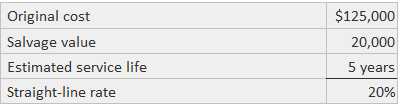Note that the double-declining multiplier yields a depreciation expense for only four years. Also, note that the expense in the fourth year is limited to the amount needed to reduce the book value to the \$20,000 salvage value.

For the 150% declining balance application, depreciation is taken over six years, with the amount in the sixth year being limited to the amount needed to produce the \$20,000 book value.

Double-declining balance depreciation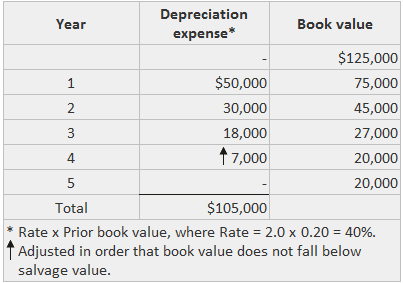150% declining balance depreciation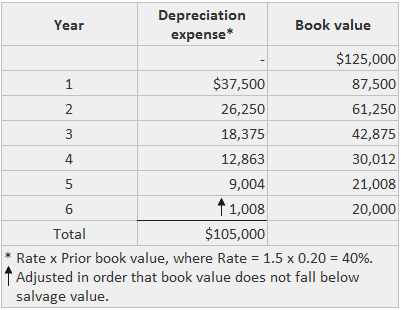### What is the declining balance method of assets depreciation?

Under the declining balance method, depreciation is charged on the book value of the asset and the amount of depreciation decreases every year.

### What is a Double-Declining Balance (DDB)?

Is a form of accelerated depreciation in which first-year depreciation is twice the amount of straight-line depreciation when a zero terminal disposal price is assumed.

### What is the 150% declining balance depreciation?

A depreciation rate equal to 1.5 times the straight-line rate.

### What are the advantages of the declining balance method?

Equal burden, simple method, suitable for tax authorities

### What are the disadvantages of the declining balance method?

No zero value, rate determination is not easy

Disclaimer: The above references an opinion and is for information purposes only. It is not intended to be investment advice. Seek a duly licensed professional for investment advice.

True is a Certified Educator in Personal Finance (CEPF®), a member of the Society for Advancing Business Editing and Writing, contributes to his financial education site, Finance Strategists, and has spoken to various financial communities such as the CFA Institute, as well as university students like his Alma mater, Biola University, where he received a bachelor of science in business and data analytics.

To learn more about True, visit his personal website, view his author profile on Amazon, his interview on CBS, or check out his speaker profile on the CFA Institute website.